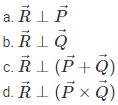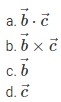# Multiplication of Vectors MCQs for NEET

NEET  Physics is the scoring paper in the medical entrance examination. Here, you will discover the NEET Physics MCQ Questions for all Concepts as per the latest syllabus. Practice more on a regular basis with these NEET Physics objective questions on air pollution and improve your subject knowledge & problem-solving skills along with time management. NEET Physics Multiplication of Vectors Multiple Choice Questions make you feel confident in answering the question in the exam & increases your scores to high.

## MCQ on Multiplication of Vectors

1. Two vectors $$2 \hat{i}+3 \hat{j}+8 \hat{k}$$ and $$4 \hat{j}-4 \hat{j}+\alpha \hat{k}$$ are perpendicular to each other. What is the value of α?
(a) ½
(b) -½
(c) 1
(d) -1

2. A force of 50 N acts on a body and displaces it through a distance of 10 meters in a direction making an angle of 600 with the force. What is the work done by the force?
(a) 100 J
(b) 150 J
(c) 200 J
(d) 250 J

3. If the sum of two vectors is perpendicular to the difference between them. The ratio of their magnitude will be
(a) 1
(b) 2
(c) 3
(d) 4

4. If $$\vec{A} \times \vec{B}=\vec{A} \cdot \vec{B}$$  then the angle between A and B will be
(a) π
(b) π/2
(c) π/3
(d) π/4

5. What is the value of $$|\vec{P} \times \vec{Q}| \text { if } \vec{P}=3 \hat{i}+\hat{j}+2 \hat{k} \text { and } \vec{Q}=2 \hat{i}-2 \hat{j}+4 \hat{k}$$?
(a) 8√3
(b) 3√8
(c) 4√3
(d) 3√4

6. If $$\vec{P} \times \vec{Q}=\vec{R}$$, then which of the following statements is true?Answer: (d) $$\vec{R} \perp(\vec{P} \times \vec{Q})$$

7. The magnitude of the scalar product of the vectors $$\overrightarrow{V_{1}}=2 \hat{i}+5 \hat{k}$$ and $$\overrightarrow{V_{1}}=2 \hat{i}+5 \hat{k}$$ is
(a) 20
(b) 36
(c) 24
(d) 34

8. Which of the following statements is true if $$\vec{P}$$  and $$\vec{Q}$$ are perpendicular to each other?Answer: (c) $$\vec{P} \cdot \vec{Q}=0$$

9. What is the angle between the vectors $$-2 \hat{i}+3 \hat{j}+\hat{k}$$ and $$\hat{i}+2 \hat{j}+-4 \hat{k}$$?
(a) 0°
(b) 25°
(c) 90°
(d) 180°

10. A force $$\vec{F}=5 \hat{i}+6 \hat{i}+4 \hat{k}$$ acts on a body and displaces it by $$\vec{S}=6 \hat{i}-5 \hat{\imath}$$. What is the work done by the force?
(a) 5 Units
(b) 10 Units
(c) 15 Units
(d) 20 Units

11. What is the area of the parallelogram which represented by vectors $$\vec{P}=2 \hat{i}+3 \hat{j}$$ and $$\vec{Q}=i+4 \hat{j}$$?
(a) 5 Units
(b) 10 Units
(c) 15 Units
(d) 20 Units

12. What is the angle between vectors $$\vec{A} \times \vec{B}$$ and $$\vec{B} \times \vec{A}$$?
(a) π
(b) 00
(c) π/2
(d) π/4

13. The angle between $$\vec{A}+\vec{B}$$ and $$\vec{A} \times \vec{B}$$ is
(a) π
(b) π/2
(c) π/4
(d) 0

14. The vectors $$\vec{a}$$, $$\vec{b}$$ and $$\vec{c}$$ satisfy the relation $$\vec{a} \cdot \vec{b}=0$$ and $$\vec{a} \cdot \vec{c}=0$$. The vector $$\vec{a}$$ is parallel toAnswer: (b)  $$\vec{b} \times \vec{c}$$